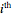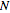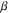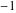# Volume 17

## Domain Coloring on the Riemann Sphere »NBCDFPDF

Published November 30, 2015

Domain coloring is a technique for constructing a tractable visual object of the graph of a complex function. The package complexVisualize.m improves on existing domain coloring techniques by rendering a global picture on the Riemann sphere (the compactification of the complex plane). Additionally, the package allows dynamic visualization of families of Möbius transformations. In this article we discuss the implementation of the package and illustrate its usage with some examples. Read More »

## Developing an Understanding of the Steps Involved in Solving Navier—Stokes Equations »NBCDFPDF

Published October 27, 2015

This article describes how Mathematica can be used to develop an understanding of the basic steps involved in solving NavierStokes equations using a finite-volume approach for incompressible steady-state flow. The main aim is to let students follow from a mathematical description of a given problem through to the method of solution in a transparent way. The well-known driven cavity problem is used as the problem for testing the coding, and the NavierStokes equations are solved in vorticity-streamfunction form. Building on what the students were familiar with from a previous course, the solution algorithm for the vorticity-streamfunction equations chosen was a relaxation procedure. However, this approach converges very slowly, so another method using matrix and linear algebra concepts was also introduced to emphasize the need for efficient and optimized code. Read More »

## Graphical Representation of Proximity Measures for Multidimensional Data »NBCDFPDF

### Classical and Metric Multidimensional Scaling

Published September 30, 2015

We describe the use of classical and metric multidimensional scaling methods for graphical representation of the proximity between collections of data consisting of cases characterized by multidimensional attributes. These methods can preserve metric differences between cases, while allowing for dimensional reduction and projection to two or three dimensions ideal for data exploration. We demonstrate these methods with three datasets for: (i) the immunological similarity of influenza proteins measured by a multidimensional assay; (ii) influenza protein sequence similarity; and (iii) reconstruction of airport-relative locations from paired proximity measurements. These examples highlight the use of proximity matrices, eigenvalues, eigenvectors, and linear and nonlinear mappings using numerical minimization methods. Some considerations and caveats for each method are also discussed, and compact Mathematica programs are provided. Read More »

## Computing Exact Closed-Form Distance Distributions in Arbitrarily Shaped Polygons with Arbitrary Reference Point »NBCDFPDF

Published June 28, 2015

We propose and implement an algorithm to compute the exact cumulative density function (CDF) of the distance from an arbitrary reference point to a randomly located node within an arbitrarily shaped (convex or concave) simple polygon. Using this result, we also obtain the closed-form probability density function (PDF) of the Euclidean distance between an arbitrary reference point and itsneighbor node whennodes are uniformly and independently distributed inside the arbitrarily shaped polygon. The implementation is based on the recursive approach proposed by Ahmadi and Pan  in order to obtain the distance distributions associated with arbitrary triangles. The algorithm in  is extended for arbitrarily shaped polygons by using a modified form of the shoelace formula. This modification allows tractable computation of the overlap area between a disk of radiuscentered at the arbitrary reference point and the arbitrarily shaped polygon, which is a key part of the implementation. The obtained distance distributions can be used in the modeling of wireless networks, especially in the context of emerging ultra-dense small cell deployment scenarios, where network regions can be arbitrarily shaped. They can also be applied in other branches of science, such as forestry, mathematics, operations research, and material sciences. Read More »

## New Symbolic Solutions of Biot’s 2D Pore Elasticity Problem »NBCDFPDF

Published May 20, 2015

This article presents new symbolic solutions for the problem of pore elasticity and pore pressure. These techniques are based on the classic theoretical approach proposed by M. A. Biot . The new symbolic solutions differ from the well-known approximations of the functions proposed for the 2D pore elasticity problem. Both new symbolic and numerical solutions are then applied to solve problems arising in offshore design technology, specifically dealing with the penetration of a gravity-based rig installed in the Arctic region of the North Sea of Russia. All symbolic approaches are based on solutions of the linear problem of the pore elasticity for homogeneous soil. The new symbolic solutions are compared with Biots solutions. Read More »

## On the Maximal Orbit Transfer Problem »NBCDFPDF

Published April 26, 2015

Assume that a spacecraft is in a circular orbit and consider the problem of finding the largest possible circular orbit to which the spacecraft can be transferred with constant thrust during a set time, so that the variable parameter is the thrust-direction angle. Also assume that there is only one center of attraction at the common center of the two circular orbits. Finally, assume normalized values for all constants and variables.

This article is divided into five sections: the orbit transfer problem, equations of motion, the optimal control problem, necessary conditions for the Mayer problem, and a dynamic approach to the maximal orbit transfer problem using Mathematicas built-in Manipulate function.

The Earth-Mars orbit transfer problem is timely, given the successful flight and smooth landing of the American Curiosity rover on Mars. Read More »

## The Quantitation of Non-classical Buffering »NBCDFPDF

### Applying the Formal and General Approach to Problems in the Biological Sciences

Published March 24, 2015

A formal, axiomatic conceptualization of buffering action—generally applicable to any physical, chemical, or biological process—was first presented by B. M. Schmitt in 2005 [1, 2]. This article provides a series of tools designed to aid in the application of these concepts to both classical and non-classical buffering phenomena. To illustrate the utility of the approach in the biological sciences, an abstract measure of the magnitude of “genetic” buffering associated with an allele of the gene encoding the heat shock protein Hsp90 is determined. Read More »

## RIFA: A Differential Gene Connectivity Algorithm »NBCDFPDF

Published February 21, 2015

This article describes the implementation of RIFA, a computational biology algorithm designed to identify the genes most directly responsible for creating differences in phenotype between two biological states, for example, tumorous liver tissue versus cirrhotic liver tissue. Read More »

## Use of Padé Approximants to Estimate the Rayleigh Wave Speed »NBCDFPDF

Published January 16, 2015

There exists a range of explicit and approximate solutions to the cubic polynomial Rayleigh equation for the speed of surface waves across an elastic half-space. This article presents an alternative approach that uses Padé approximants to estimate the Rayleigh wave speed with five different approximations derived for two expansions about different points. Maximum relative absolute errors of between 0.34% and 0.00011% occur for the full range of the Poisson ratio fromto 0.5. Even smaller errors occur when the Poisson ratio is restricted within a range of 0 to 0.5. For higher-order approximants, the derived expressions for the Rayleigh wave speed are more accurate than previously published solutions, but incur a slight cost in extra arithmetic operations, depending on the desired accuracy. Read More »